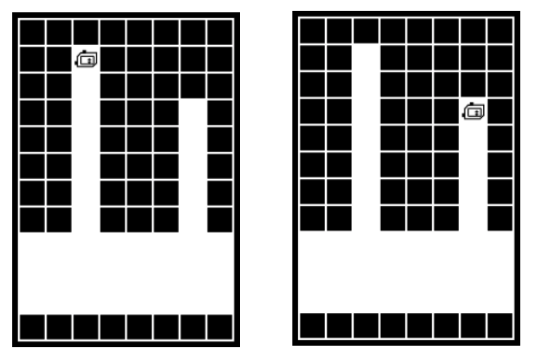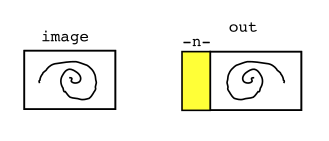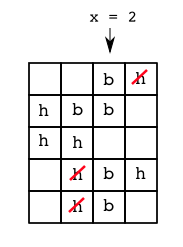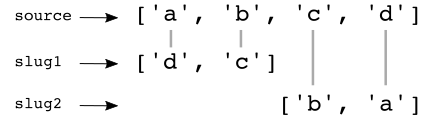### Instructions

• There are 6 problems to complete
• In Bluebook, "Problem 1" is these instructions and reference code
• Problems 2-7 are the actual problems
• This is a closed note exam
• The exam is 60 minutes and 100 points, graded on a curve
• We will not grade off for syntax errors, so long as we see the correct idea
• We will not grade off if you omit the final "return" at the end of a function, so long as the code computes the correct result
• Good luck!
```# CS106A Exam Reference Reminder
# [square brackets] denote functions listed here that we have not used yet
# Exam questions will not depend on functions we have not used yet
Bit:
bit = Bit(filename)
bit.front_clear() bit.left_clear() bit.right_clear()
bit.move() bit.left() bit.right()
bit.paint('red') [bit.erase()]

General functions:
len() int() str() range() [list() sorted()]
String functions:
isalpha() isdigit() isspace() isupper() islower()
find() upper() lower() [strip()]
List functions:
append() index() [extend() pop()  insert()]

SimpleImage:
image = SimpleImage(filename)
# create blank image
image = SimpleImage.blank(width, height)

# foreach loop
for pixel in image:

# range/y/x loop
for y in range(image.height):
for x in range(image.width):
pixel = image.get_pixel(x, y)

Grid 2D:
grid = Grid.build([['row', '0'], ['row', '1']])
grid.width, grid.height - properties
grid.in_bounds(x, y) - True if in bounds
grid.get(x, y) - returns contents at that x,y, or None if empty
grid.set(x, y, value) - sets new value into grid at x,y

Read lines out of a text fie:
with open(filename) as f:
for line in f:
```

### Warmup Trace Problem

a. For each ??, write what value comes from the Python expression on each line.

b. What 3 numbers print when the caller() function runs? This code runs without error.

```Part a:

>>> 2 - 1 + 3
4
>>>
>>> 1 + 2 * 3
7
>>>
>>> 7 // 2
3
>>>
>>> s = 'Dune'
>>> s
'e'
>>>
>>> s[:2]
'Du'
>>>
>>> s[2:]
'ne'

Part b:

def foo(a)
b = a + 2
return b

def caller():
a = 5
b = 6
c = foo(b)
print(a, b, c)

# Write 3 numbers here
5 6 8

```

### 3. Bit (10 points)

Bit is living like a bat, facing down at the top of a vertical shaft. Move bit down, just out of the shaft. Move bit towards the right side of the world, where there is a second shaft going up. Move bit up to the top of the second shaft, ending facing down as at the start. Your code should work for any pair of shafts, regardless of their size of spacing.

Bit before and after:```def bitbat(filename):
bit = Bit(filename)
# move to bottom of shaft
while not bit.left_clear():
bit.move()

# find second shaft
bit.left()
bit.move()
while not bit.left_clear():
bit.move()

# move up to the top
bit.left()
while bit.front_clear():
bit.move()

# end facing down
bit.left()
bit.left()
```

### 4. Image (15 points)

Given an image filename and int n which is 1 or more. Create a new out image the same height as the original with an n-pixel-wide vertical yellow stripe running down its left side, like on the homework. Add a horizontally flipped copy of the original image to the right of the stripe. The starter code includes an a-b-c outline and some code you can use, but any solution which gets the right output is fine.```def reverse_yellow(filename, n):
image = SimpleImage(filename)
# a. Create out image
out = SimpleImage.blank(image.width + n, image.height)

# b. Loop over original image, write flipped copy
for y in range(image.height):
for x in range(image.width):
pixel = image.get_pixel(x, y)
out_pix = out.get_pixel(out.width - x - 1, y) # out_pix = out.get_pixel(image.width - x - 1 + n, y)
out_pix.red = pixel.red
out_pix.green = pixel.green
out_pix.blue = pixel.blue

# c. Create stripe
for y in range(out.height):
for x in range(n):
out_pix = out.get_pixel(x, y)
out_pix.blue = 0

return out
```

### 5. Grid (25 points)

There are two functions for this problem, and you can get full credit for each function independent of what you do on the other function.

Bears love honey. We have a Grid representing Yosemite national park, and every square is either a bear `'b'`, honey `'h'`, a tree `'t'`, or empty `None`.

a. honey_dx(grid, x, y): Given a grid and an in bounds x,y. If the square contains a bear, do the following: if the square immediately to the left contains honey, return -1. If the left does not contain honey, but the square to the right contains honey, return 1. In all other cases - no bear or no honey - return 0. So in effect, a 1 or -1 return value indicates that there's a bear, and the x direction of some honey.

b. feed_column(grid, x): Given a grid and an in-bounds x value. The x value defines a column within the grid. This function feeds all the bears in the column. Do the following for every square in the column: Check each square by calling the the honey_dx() helper function. If the square contains a bear and there is honey to its left or right, set the honey square to `None` - in effect the bear eats it. If there is honey on both sides, eat the left side. Return the changed grid.```def honey_dx(grid, x, y):
if grid.get(x, y) != 'b':
return 0

if grid.in_bounds(x - 1, y) and grid.get(x - 1, y) == 'h':
return -1

if grid.in_bounds(x + 1, y) and grid.get(x + 1, y) == 'h':
return 1

return 0

def feed_column(grid, x):
for y in range(grid.height):  # this a stretch?
dx = honey_dx(grid, x, y)  # this part seems pretty straightforward
# the muggle way -1/+1 - fine
if dx == -1:
grid.set(x - 1, y, None)
if dx == 1:
grid.set(x + 1, y, None)
# the cool way
# if dx != 0:
#     grid.set(x + dx, y, None)  # nom nom nom nom
return grid

```

### 6. Short Strings (15 points)

Write code for the two functions:

`double_digit(s):` Given string s. Return a string made of two copies of each digit char in s, so `'42ab3\$'` returns `'442233'`

`sum_digits(s):` If there is a `'#'` in s, it is guaranteed that there will be exactly two `'#'` in s and each will be immediately followed by a digit. Return the arithmetic sum of the two `'#'` digits, or 0 if there is no `'#'`. So `'3x#4abc#8xxx'` returns `12`

```def double_digit(s):
result = ''
for ch in s:
if ch.is_digit():
result += ch + ch
return result

def sum_digits(s):
hash_loc_first = s.find('#')
if hash_loc_first == -1:
return 0

first_dig = s[hash_loc_first + 1]

hash_los_sec = s.find('#', hash_loc_first + 1)

second_dig = s[hash_loc_sec + 1]

return int(first_dig) + int(second_dig)
```

### 7. Double Slug Decryption (25 points)

First we will describe the double-slug encryption system used in this problem, then you will write the decrypt function below. As a simplification, we will assume that uppercase chars do not exist for this problem.

Double-slug encryption uses one source list and two slug lists, all containing lowercase chars. The source list is even length, and the two slugs are each half its length. The slug1 list holds the encrypted form of chars in the first half of the source list. The slug2 list holds the encrypted form of chars in the second half of the source list. No char is in both slug1 and slug2. Here is an example with a length-4 source list:Encrypt examples with source/slugs above:

```The encrypt of 'a' is 'd'
The encrypt of 'c' is 'b'
```

Write the code for the decrypt function: Given the lists of lowercase chars: source, slug1, slug2. And given lowercase ch which is encrypted if possible. Return the decrypted form of ch, or return ch unchanged if it is not encrypted (e.g. `'\$'`). The index in the source list where the slug2-encryption begins is at midway = len(source) // 2, which is included in the starter code.

Decrypt examples with source/slugs above:

```The decrypt of 'd' is 'a'
The decrypt of 'b' is 'c'
```
```def decrypt(source, slug1, slug2, ch):
midway = len(source) // 2
pass
if ch in slug1:
idx = slug1.index(ch)
return source[idx]
if ch in slug2:
idx = slug2.index(ch)
return source[midway + idx]
return ch

```